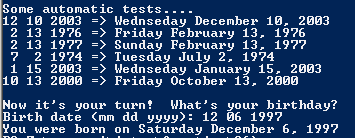# Assignemnt #96

## Code

```///colin hinton
///5th period
///WeekdayCalc.java
///1/6/2015

import java.util.Scanner;

public class WeekdayCalc
{
public static void main( String[] args )
{
Scanner keyboard = new Scanner(System.in);

System.out.println("Welcome to Mr. Davis's fantastic birth-o-meter!");
System.out.println();
System.out.println("All you have to do is enter your birth date, and it will");
System.out.println("tell you the day of the week on which you were born.");
System.out.println();
System.out.println("Some automatic tests....");
System.out.println("12 10 2003 => " + weekday(12,10,2003));
System.out.println(" 2 13 1976 => " + weekday(2,13,1976));
System.out.println(" 2 13 1977 => " + weekday(2,13,1977));
System.out.println(" 7  2 1974 => " + weekday(7,2,1974));
System.out.println(" 1 15 2003 => " + weekday(1,15,2003));
System.out.println("10 13 2000 => " + weekday(10,13,2000));
System.out.println();

System.out.print("Birth date (mm dd yyyy): ");
int mm = keyboard.nextInt();
int dd = keyboard.nextInt();
int yyyy = keyboard.nextInt();

System.out.println("You were born on " + weekday(mm,dd,yyyy));
}

public static String weekday( int mm, int dd, int yyyy )
{
int yy, total;
String date = "";
yy = yyyy - 1900;
total = (yy / 4) + yy + dd;
total = monthOffset(mm) + total;
boolean leapyear = isLeap(yyyy);
if (leapyear == true )
{
if (mm == 1 || mm == 2 )
total = total - 1;

}

int weekday = total%7;
weekday_name(weekday);

date = weekday_name(weekday) + " " + monthName(mm) + " " + dd + ", " + yyyy;

return date;
}

public static String monthName( int mm)
{
String ans;
if ( mm == 1 )
ans = "January";
else if ( mm == 2 )
ans = "February";
else if ( mm == 3 )
ans = "March";
else if ( mm == 4 )
ans = "April";
else if ( mm == 5 )
ans = "May";
else if ( mm == 6 )
ans = "June";
else if ( mm == 7 )
ans = "July";
else if ( mm == 8 )
ans = "August";
else if ( mm == 9 )
ans = "September";
else if ( mm == 10 )
ans = "October";
else if ( mm == 11 )
ans = "November";
else if ( mm == 12 )
ans = "December";
else
ans = "Error";

return ans;
}
public static int monthOffset( int mm )
{
int result;
if (mm == 1)
result = 1;
else if (mm == 2)
result = 4;
else if (mm == 3)
result = 4;
else if (mm == 4)
result = 0;
else if (mm == 5)
result = 2;
else if (mm == 6)
result = 5;
else if (mm == 7)
result = 0;
else if (mm == 8)
result = 3;
else if (mm == 9)
result = 6;
else if (mm == 10)
result = 1;
else if (mm == 11)
result = 4;
else if (mm == 12)
result = 6;
else
result = -1;
return result;
}
public static String weekday_name( int weekday )
{
String result = "";

if ( weekday == 1 )
{
result = "Sunday";
}
else if ( weekday == 2 )
{
result = "Monday";
}
else if(weekday == 3)
{
result = "Tuesday";
}
else if(weekday == 4)
{
result = "Wednseday";
}
else if(weekday == 5)
{
result = "Thursday";
}
else if(weekday == 6)
{
result = "Friday";
}
else if(weekday == 7)
{
result = "Saturday";
}
else if(weekday == 0)
{
result = "Saturday";
}
else
{
result = "error";
}
return result;

}

public static boolean isLeap( int yyyy )
{
boolean result;

if ( yyyy%400 == 0 )
{
result = true;
}
else if ( yyyy%100 == 0 )
{
result = false;
}
else if ( yyyy%4 == 0 )
{
result = true;
}
else
{
result = false;
}

return result;
}
}
```

### Picture of the output